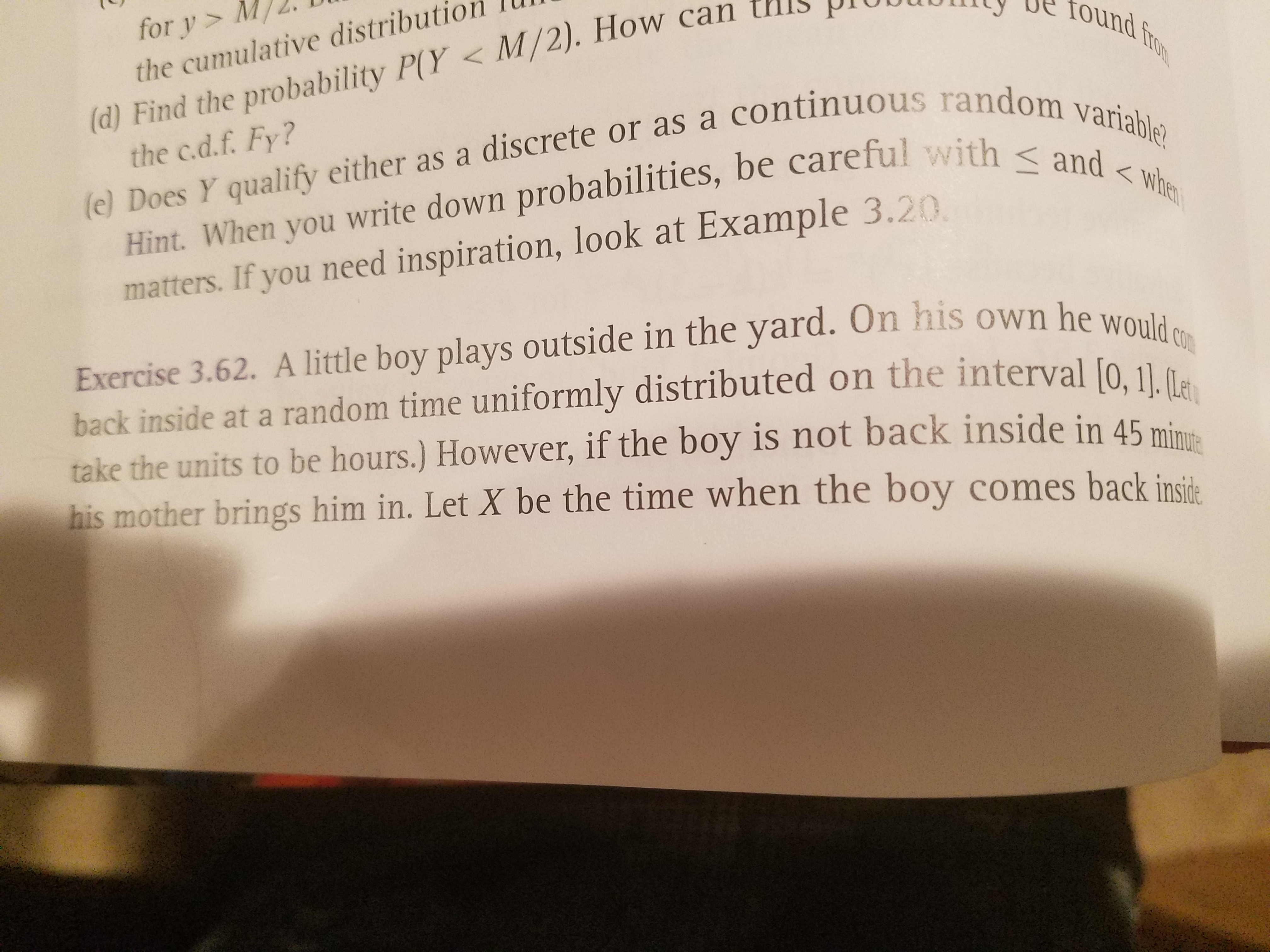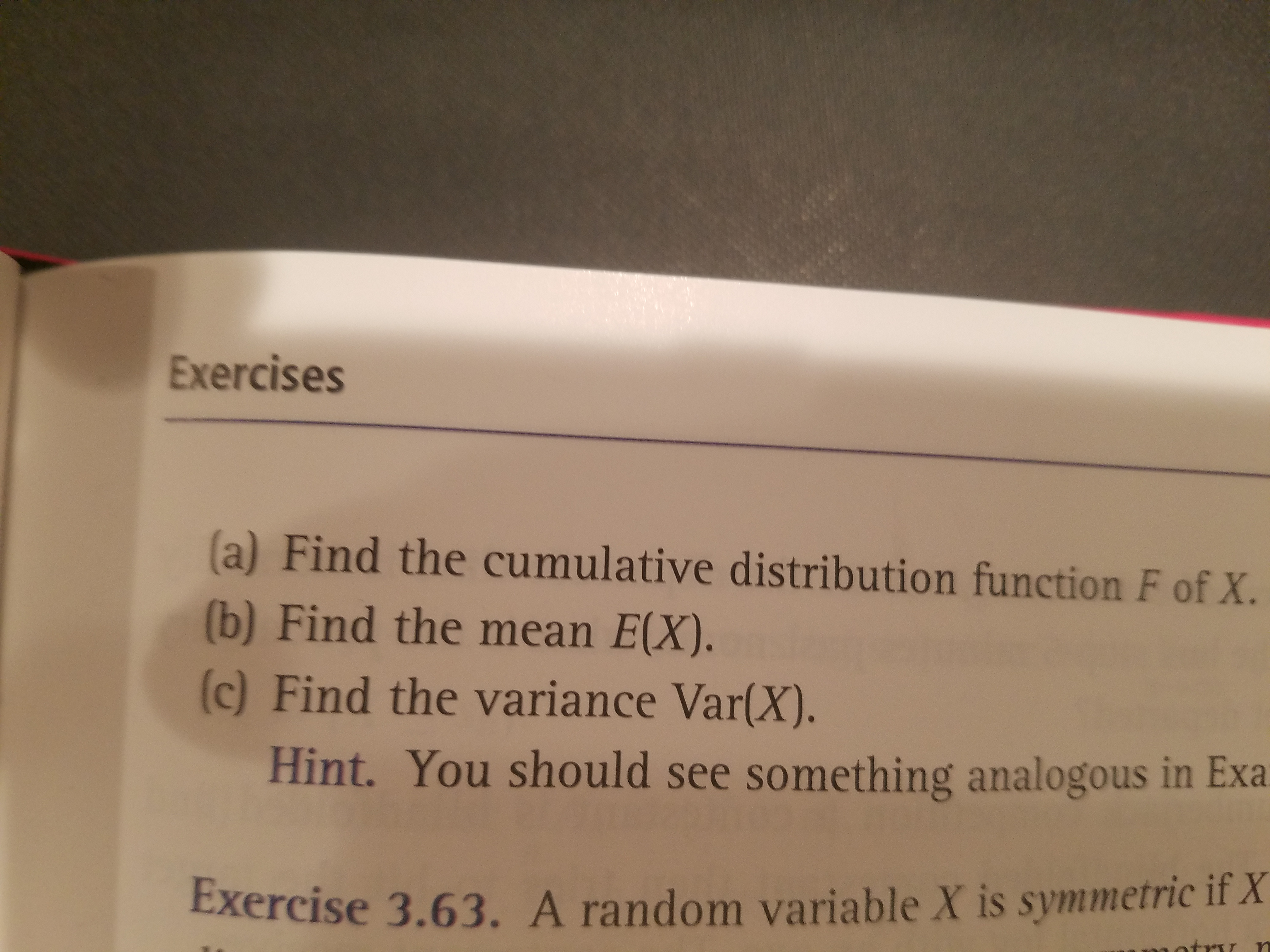# for y> M/the cumulative distribution(d) Find the probability P(Y < M/2). How can tlllthe c.d.f. Fy?found(e) Does Y qualify either as a discrete or as a continuous random variableHint. When you write down probabilities, be careful with and

Questionhelp_outlineImage Transcriptionclosefor y> M/ the cumulative distribution (d) Find the probability P(Y < M/2). How can tlll the c.d.f. Fy? found (e) Does Y qualify either as a discrete or as a continuous random variable Hint. When you write down probabilities, be careful with andhelp_outlineImage TranscriptioncloseExercises a) Find the cumulative distribution function F of X. (b) Find the mean E(X). (c) Find the variance Var(X). Hint. You should see something analogous in Exa Exercise 3.63. A random variable X is symmetric if X fullscreen
check_circleExpert Solution
Step 1

Introduction:

Some properties of the continuous uniform distribution:

Probability density function (pdf):

If X ~ U (a, b), the pdf f (x) = 1/(ba); a < X < b.

Cumulative distribution function (cdf):

The cdf F (x) = (xa)/(ba); a < x < b.

Expectation:

The mean or expectation of X is, E (X) = (a...

### Want to see the full answer?

See Solution

#### Want to see this answer and more?

Solutions are written by subject experts who are available 24/7. Questions are typically answered within 1 hour*

See Solution
*Response times may vary by subject and question
Tagged in

### Random Variables